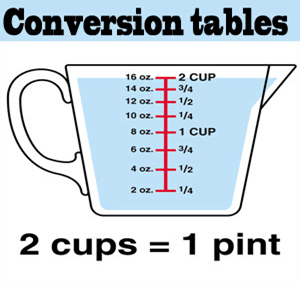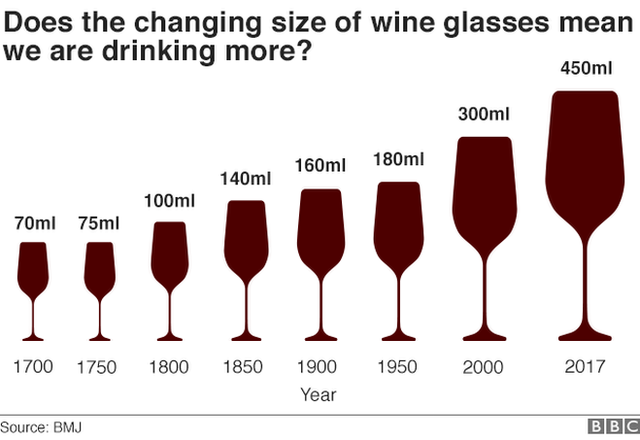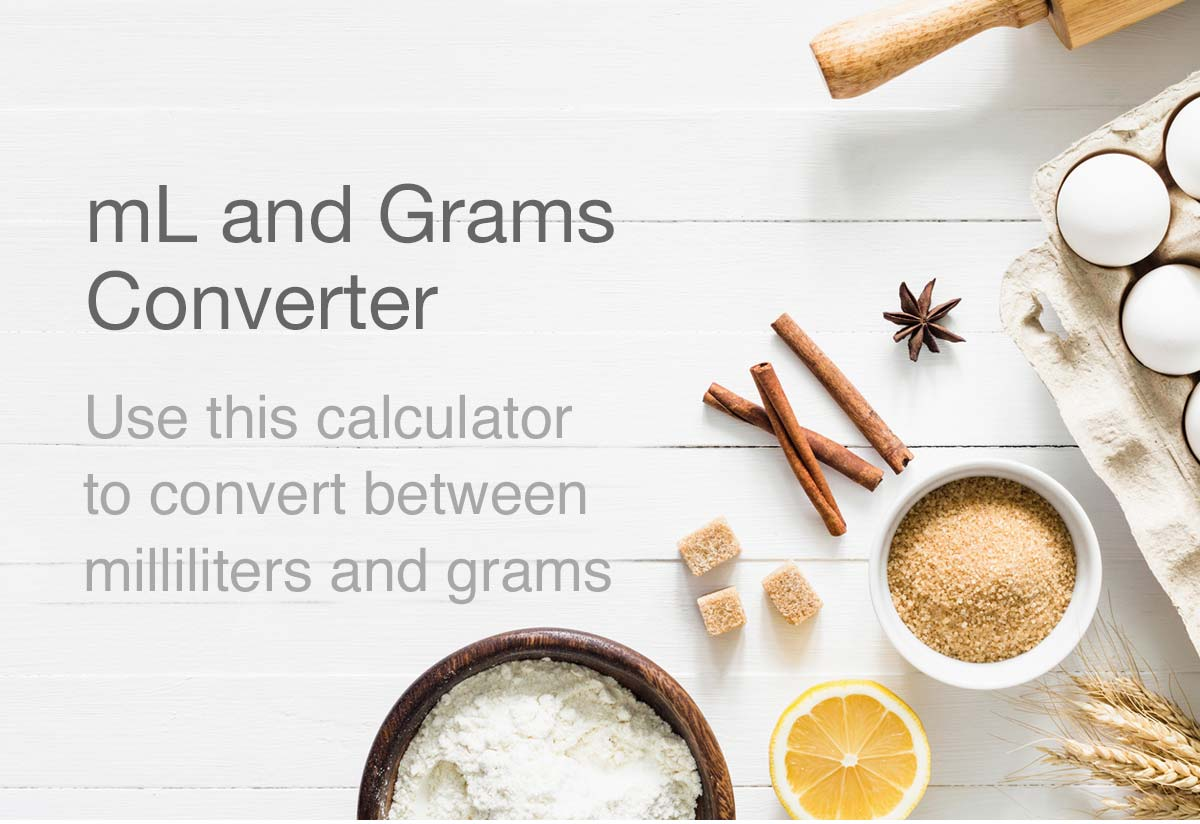# How many mL in a cup? The complete guide

Since childhood, studying quantities has been an integral part of our studies.

Even while watching some baking videos on YouTube, we have been asked to add 3-4 cups of milk into our cake batter. The question that arises is ‘How many mL in a cup?’ Consequently, we always try to convert these measurements in cups to mL.

Generally for defining these quantities, we specify them in units. Since these units are acceptable worldwide, people can understand their meaning easily. To specify, milliliter is one such unit of measurement. However, we use it to specify quantities of fluids. Gasses and liquids are referred to as fluids.## What is a milliliter?

A milliliter is a unit of volume for liquids and gasses that is equal to a thousandth of a liter.

Indeed, it is a very small quantity. In fact, a milliliter would not even be enough water to fill up a small spoon – you’d still be very thirsty! A milliliter is a unit used to measure capacity. Also, it is equal to one-thousandth of a liter. In other words, 1,000 milliliters could fit inside a one-liter container.

## How many mL in a cup UK?

Often, we need to convert kitchen culinary volume and capacity measuring units from ml to how many cups. Thus, we face the question “How many mL in a cup?”

The UK cups are 250 mLs and US cups are 240 mLs, so UK half cups are 125 mLs whereas US half cups are 120mLs (quarter cups tend to be the same at 60 mLs).

Actually, these measurements come quite handy while we need to be accurate with our measurements. For example, while baking we need to make sure to add the right amount of milk, or cocoa powder into our batter.

Infact, we need to convert the volume of most liquids (water, juice, milk, cream) from imperial to metric:

 In cups In ml 1 cup 250 mL ¾ cup 175 mL ½ cup 125 mL 1⁄3 cup 80 mL ¼ cup 60 mL 2 Tbsp 30 mL

This table represents the value of cups in milliliters for most liquids.## How many mL in a cup of water?

For instance, when we measure water in a cup, we do that using a US cup measure.

So, the cup is a cooking measure of volume, commonly associated with cooking and serving sizes. However, it is traditionally equal to one-half US pint (236.6 mL). Because actual drinking cups may differ greatly from the size of this unit, standard measuring cups may be used, with a metric cup being 250 milliliters.

Therefore, a US cup can hold 236.588 milliliter. So, to get an approximate result, you can multiply the volume value by 237.

## How many mL in a cup of medicine?

For example, there are certain measures in the context of nursing and medicines. Also, these measures have to be very accurate. So, it is a very crucial step.

Here, the table below demonstrates the conversion of certain medical instruments to ounces, and finally to milliliter:

 1 tbsp. 3 tsp. 1 fl. oz. 2 tbsp. 1 cup 8 fl. oz. 1 tsp. 5 mL 1 tbsp. 15 mL 1 fl. oz. 30 mL 1 cup 250 mL 1 L 1000 mL

## How many mL in a cup of milk?

Just as the measure of water, milk can also be measured in US or UK cups quite easily. Since a US cup is roughly 237 milliliters, that cup can hold near about 237 milliliters of milk. And, half a cup of milk contains around 118 milliliters of milk. On the contrary, a UK cup is 250 mL. Hence, it will contain around 250 mL of milk.

## How many mL in a cup of coffee?

Coffee cups come in a variety of sizes, usually suited to the type of beverage you are having.Some coffee chains have their own fancy nicknames for sizes, too(I’m looking at you, Starbucks!). But what are standard coffee cup sizes?

For example, the cup size of an espresso shot is 2 ounces and it holds about 59 mL.

Furthermore, the cup size of a latte is 8 ounces and it holds about 236 mL.

Also, the cup size of an espresso shot is 12 ounces and it holds about 355 mL.

As you can see, a “standard” coffee cup size can be considered as 6 to 8 ounces. That’s big enough for typical brewed coffee and cappuccinos.

However, standards are not international. Since the USA is the only country aside from Liberia and Myanmar to use the Imperial measurement system, 8 ounces is not accepted everywhere. Read Also: Height Conversion Chart of All Units | Inches to Meters

Most countries that use the metric system choose the nice round number of 250 ml as a standard cup size. Here’s the kicker: 250 ml is 8.4 ounces!

The size of your cup is important because it dictates how much coffee you should use to brew it.

If you brew 12 ounces of liquid coffee with just 15 grams of coffee grounds, you’ll get a really weak brew, since 15 ounces is what’s used for the average coffee cup size of 6 ounces.So to get a proper 12 ounce brew, you’ll need double the coffee. What’s more, using double the coffee means you’ll be ingesting double the caffeine!## How many mL in a cup in Australia?

Truly, precise measurements can be subsequently vital to cooking and baking success. However, in Australia, you will find Australian Standard measuring cups and spoons.

Also, here is a list displaying Australian cup and spoon sizes in milliliters:

## For solids:

 Cup Metric 1/3 cup 80ml 1/2 cup 125ml 1 cup 250ml 1/4 cup 60ml Spoon Metric 1/4 teaspoon 1.25ml 1/2 teaspoon 2.5ml 1 teaspoon 5ml 2 teaspoons 10ml 1 tablespoon (equal to 4 teaspoons) 20ml

## How many ml in a cup of wine?

Meanwhile in our daily lives, we do not pay any attention to the size of cups.

However, the standard pour for a glass of wine is five ounces, or 150 milliliters.

Also, it is typically the one bars and restaurants use when they serve you a glass of vino with dinner.

But when you’re home and left to your own devices, the pours may tip a bit taller than any official standard.

However, that may be because the wine glass you’re using just keeps getting bigger.

Moreover, a new study from researchers at England’s University of Cambridge reveals that the average wine glass has grown subsequently to sevenfold in the past 300 years.

Further, researchers say the increase in the size of wine glasses may be partly to blame for the increase in drinking. Though, there’s marketing and affordability, too.### How many mL in a cup of flour?

Accordingly, unit symbols used by international culinary educational institutions and training for these two plain flour (PF) white measures are:

Thus, prefix or abbreviation ( abbr. ) short brevis unit symbol for US cup is: us cup

Thus, prefix or abbreviation ( short abbr. brevis ) unit symbol for milliliter is: mL.

How many milliliters of plain flour (PF) white are in 1 US cup?

The answer is: The change of 1 us cup ( US cup ) unit in a plain flour (PF) white measure equals 236.59 ml ( milliliter ) as per the equivalent measure and for the same plain flour (PF) white type.

In fact, professional people always ensure, and their success in fine cooking also depends on them getting the most precise unit conversion results in measuring their ingredients. Likewise, in speciality cooking a measure of plain flour (PF) white can be crucial. If there is an exact measure in a US cup – US cups for plain flour (pf) white, it’s the rule in culinary career, that the US cup portion number gets converted into ml – milliliters of plain flour (PF) white absolutely exactly. In brief, it’s like an insurance for the master chef for always having all the meals created perfectly.### Unit converters:

Often we need to convert units in our day-to-day calculations. In fact, we use them greatly in case of finding length, breadth, area, perimeter, and volumes of figures. Similarly, we might often have to convert mL to various other units.

Below, we are going to discuss many of these converters one by one.

### mL = gm

In the same way, the conversion from mL to grams is actually quite simple.

Indeed, it simply follows the proportion 1:1. So, it becomes quite simple to convert mL to grams( gm ).

For example, 1 mL = 1 gm or 500 mL = 500 gm, for that matter.

Also, you can easily access any online calculator that can convert mL to grams.

Here, we are providing you the link for one such calculator:

mL to gm converter### mL = l

A milliliter (symbol: mL) is a unit of volume that is accepted for use in the international system of units (SI). Specifically, one milliliter is equal to 1 cubic centimeter (cm3), 1/1,000,000 cubic meters (m3), or 1/1000 liters.

However, the base unit of the milliliter is the liter (US spelling), spelled “liter” in SI terms. Additionally, the term “liter” was originally part of the French metric system and was derived from the term “litron,” one of the older versions of the French liter.

1 mL = 0.001 L,

1 L = 1000 mL

For example: convert 15 mL to L,

Or, 15 mL = 15 × 0.001 L, l = 0.015 L.

However, this calculator allows you to convert mL to L automatically:

mL to L converter

### mL = cc

For instance, mL and cc are the same measurement; there is no difference in volume.

However, the primary difference is that milliliters are used for fluid amounts while cubic centimeters are used for solids. But, no matter what is being measured, 1 cc always equals 1 mL (1 ml = 1 cc).

Also, the cubic centimeter is used in many scientific disciplines, often in the form of milliliters (symbol: mL). Although the cubic centimeter is based on the SI unit of volume, the mL (and its multiples) are more commonly used as a measurement of volume than the cubic centimeter.

However, the United States medical and automotive fields are an exception to this, and the cubic centimeter is still widely used in these fields. On the contrary, the United Kingdom uses cubic centimeters in the medical, but not the automotive field.

Here, this calculator converts mL to cc very easily:

mL to cc converter

### mL = kg

Namely, mL is the SI unit of volume and kg is a unit of mass.

However, volume and mass do not have a direct relationship.

Further, to convert the volume of any object to its weight, multiply the volume of the object by the density. Now, our ml to kg converter easily converts any object volume to weight in a single click.

To illustrate, Volume x Density = Mass

A milliliter is an international standard unit in a metric system. Also, a milliliter is a measuring unit of volume and is abbreviated as mL or ml. Milliliter is referred to as mL. Finally, a kilogram is a standard unit in a metric unit system. Additionally, a kilogram is a measuring unit of mass and is abbreviated as kg.

Consequently, one kg is approximately equal to 1000 ml.

Finally, click on this link to access the mL to kg calculator:

mL to kg calculator

### mL = mg

Moreover, to convert a milliliter measurement to a milligram measurement, multiply the volume by 1,000 times the density of the ingredient or material.

Moreover, you can use this simple formula to convert:

milligrams = milliliters × 1,000 × ingredient density

Thus, the volume in milligrams is equal to the milliliters multiplied by 1,000 times the density of the ingredient or material.

For example, here’s how to convert 5 milliliters to milligrams for an ingredient with a density of 0.7 g/mL.

5 ml = 5 × 1,000 × 0.7

Or, 3,500 mg.

Now, this calculator will easily help you to convert mL to mg:

mL to mg converter## How many mL in a cup FAQ’s

### 1.   Is 250 mL equal to one cup?

Ans: Since, volume of most liquids (water, juice, milk, cream) are converted by volume from imperial to metric: So, 1 cup = 250 mL. ¾ cup = 175 mL. ½ cup = 125 mL.

### 2.   How many mL in a cup in Canada?

Ans: Currently, Canada usually employs the metric cup of 250 ml, but its conventional cup was somewhat smaller than both American and imperial units.

### 3.   How much is 225 gram flour in cups?

Ans: On the whole, 225 gram flour in cups is equivalent to 7.9 ounces (oz.).

### 4.   How many cups is 500 mL in Canada?

Ans: Currently, in Canada, 2.25 cups is equivalent to 500 mL.

### 5.   What size in an Australian cup?

Ams: However, there is a difference between US and Australian cup measures -on one hand, a US cup is 240ml and on the other hand, an Australian cup is 250 mL.

### 6.   How many mL is a quart in Canada?

Ans: According to the metric system, one quart is equivalent to one liter, or 1000 mL.

### 7.   How much does one cup of flour weigh in Australia?

Ans: Currently, in Australia, one cup of flour weighs 150 grams.

### 8.   What is a 100 gram Aus cup?

Ans: In brief, one – 100 grams of plain flour (PF) white converted to Australian cup equals 0.76 Au. cup.

### 9.   Where are mL measurements commonly used?

Ans: For instance, Cosmetics, toothpaste, deodorants, shampoo are perhaps the commonest. Besides these, small quantities of liquid or paste foodstuffs are also measured in milliliters. However, most of the world will call these milliliters the correct international spelling. A “standard” water glass is 170 ml, a tea cup is 250 ml, a beer mug can be 330 or 500 ml.